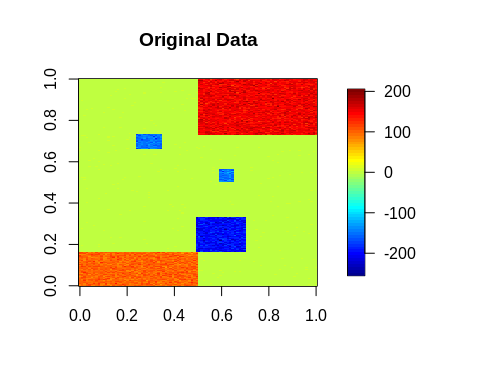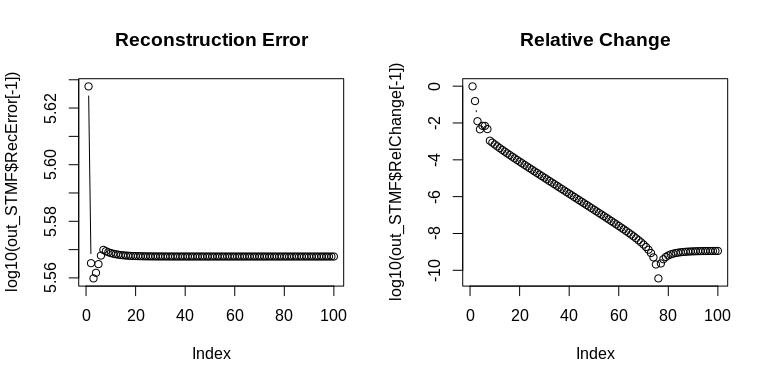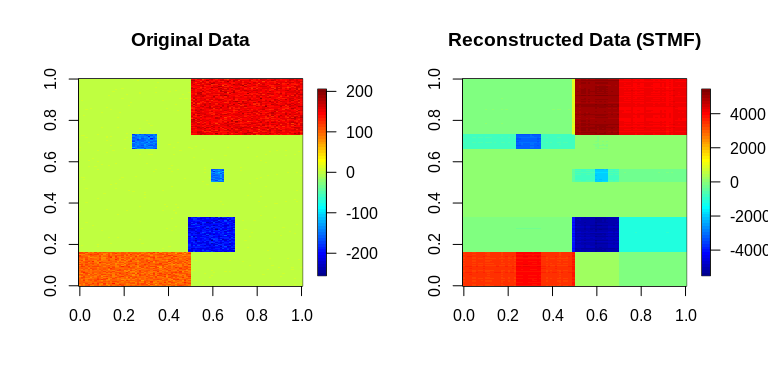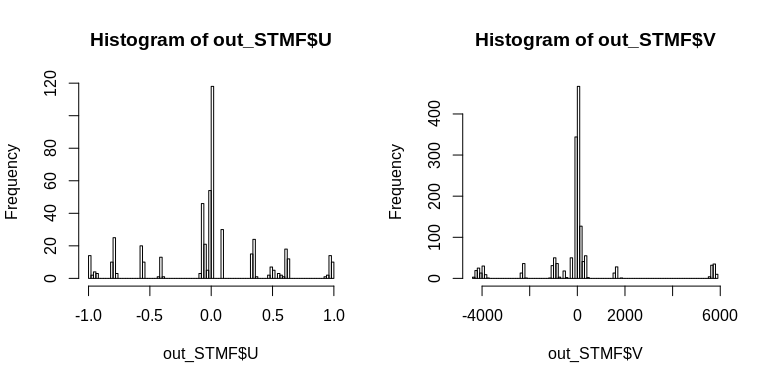# 2. Discretized Singular Value Decomposition (dSVD)

Laboratory for Bioinformatics Research, RIKEN Center for Biosystems Dynamics Research

# Introduction

In this vignette, we consider approximating a matrix as a product of two low-rank matrices (a.k.a., factor matrices).

Test data is available from toyModel.

library("dcTensor")
X <- dcTensor::toyModel("dSVD")

You will see that there are five blocks in the data matrix as follows.

suppressMessages(library("fields"))
image.plot(X, main="Original Data", legend.mar=8)# Semi-Ternary Matrix Factorization (STMF)

Here, we introduce the ternary regularization to take {-1,0,1} values in $$U$$ as below:

$X \approx U V' \ \mathrm{s.t.}\ U \in \{-1,0,1\},$ where $$X$$ ($$N \times M$$) is a data matrix, $$U$$ ($$N \times J$$) is a ternary score matrix, and $$V$$ ($$M \times J$$) is a loading matrix. In dcTensor package, the object function is optimized by combining gradient-descent algorithm (Tsuyuzaki 2020) and ternary regularization.

## Basic Usage

In STMF, a rank parameter $$J$$ ($$\leq \min(N, M)$$) is needed to be set in advance. Other settings such as the number of iterations (num.iter) are also available. For the details of arguments of dSVD, see ?dSVD. After the calculation, various objects are returned by dSVD. STMF is achieved by specifying the ternary regularization parameter as a large value like the below:

set.seed(123456)
out_STMF <- dSVD(X, Ter_U=1E+10, J=5)
str(out_STMF, 2)
## List of 6
##  $U : num [1:100, 1:5] 0.00592 0.00582 0.00626 0.00641 0.00611 ... ##$ V            : num [1:300, 1:5] 89.8 94.8 93.6 101 87.6 ...
##  $RecError : Named num [1:101] 1.00e-09 4.24e+05 3.67e+05 3.63e+05 3.65e+05 ... ## ..- attr(*, "names")= chr [1:101] "offset" "1" "2" "3" ... ##$ TrainRecError: Named num [1:101] 1.00e-09 4.24e+05 3.67e+05 3.63e+05 3.65e+05 ...
##   ..- attr(*, "names")= chr [1:101] "offset" "1" "2" "3" ...
##  $TestRecError : Named num [1:101] 1e-09 0e+00 0e+00 0e+00 0e+00 0e+00 0e+00 0e+00 0e+00 0e+00 ... ## ..- attr(*, "names")= chr [1:101] "offset" "1" "2" "3" ... ##$ RelChange    : Named num [1:101] 1.00e-09 9.70e-01 1.55e-01 1.25e-02 4.53e-03 ...
##   ..- attr(*, "names")= chr [1:101] "offset" "1" "2" "3" ...

The reconstruction error (RecError) and relative error (RelChange, the amount of change from the reconstruction error in the previous step) can be used to diagnose whether the calculation is converged or not.

layout(t(1:2))
plot(log10(out_STMF$RecError[-1]), type="b", main="Reconstruction Error") plot(log10(out_STMF$RelChange[-1]), type="b", main="Relative Change")The product of $$U$$ and $$V$$ shows whether the original data is well-recovered by dSVD.

recX <- out_STMF$U %*% t(out_STMF$V)
layout(t(1:2))
image.plot(X, main="Original Data", legend.mar=8)
image.plot(recX, main="Reconstructed Data (STMF)", legend.mar=8)The histograms of $$U$$ and $$V$$ show that $$U$$ looks ternary but $$V$$ does not.

layout(t(1:2))
hist(out_STMF$U, breaks=100) hist(out_STMF$V, breaks=100)# Session Information

## R version 3.6.3 (2020-02-29)
## Platform: x86_64-conda-linux-gnu (64-bit)
## Running under: CentOS Linux 7 (Core)
##
## Matrix products: default
## BLAS/LAPACK: /home/koki/miniconda3/lib/libopenblasp-r0.3.17.so
##
## locale:
##   LC_CTYPE=en_US.UTF-8       LC_NUMERIC=C
##   LC_TIME=en_US.UTF-8        LC_COLLATE=en_US.UTF-8
##   LC_MONETARY=en_US.UTF-8    LC_MESSAGES=en_US.UTF-8
##   LC_PAPER=en_US.UTF-8       LC_NAME=C
##  LC_MEASUREMENT=en_US.UTF-8 LC_IDENTIFICATION=C
##
## attached base packages:
##  stats     graphics  grDevices utils     datasets  methods   base
##
## other attached packages:
##  nnTensor_1.1.12   fields_13.3       viridis_0.6.2     viridisLite_0.4.0
##  spam_2.8-0        dcTensor_1.0.1
##
## loaded via a namespace (and not attached):
##   Rcpp_1.0.8         highr_0.9          RColorBrewer_1.1-2 rTensor_1.4.8
##   bslib_0.3.1        compiler_3.6.3     pillar_1.7.0       jquerylib_0.1.4
##   tools_3.6.3        dotCall64_1.0-1    digest_0.6.29      jsonlite_1.8.0
##  evaluate_0.15      lifecycle_1.0.1    tibble_3.1.2       gtable_0.3.0
##  pkgconfig_2.0.3    rlang_0.4.11       DBI_1.1.2          yaml_2.3.5
##  xfun_0.29          fastmap_1.1.0      gridExtra_2.3      stringr_1.4.0
##  dplyr_1.0.6        knitr_1.37         generics_0.1.2     sass_0.4.0
##  vctrs_0.3.8        maps_3.4.0         plot3D_1.4         tidyselect_1.1.1
##  grid_3.6.3         glue_1.4.2         R6_2.5.1           fansi_1.0.2
##  tcltk_3.6.3        rmarkdown_2.11     purrr_0.3.4        ggplot2_3.3.5
##  magrittr_2.0.2     scales_1.1.1       htmltools_0.5.2    ellipsis_0.3.2
##  MASS_7.3-55        tagcloud_0.6       misc3d_0.9-1       assertthat_0.2.1
##  colorspace_2.0-3   utf8_1.2.2         stringi_1.7.6      munsell_0.5.0
##  crayon_1.5.0